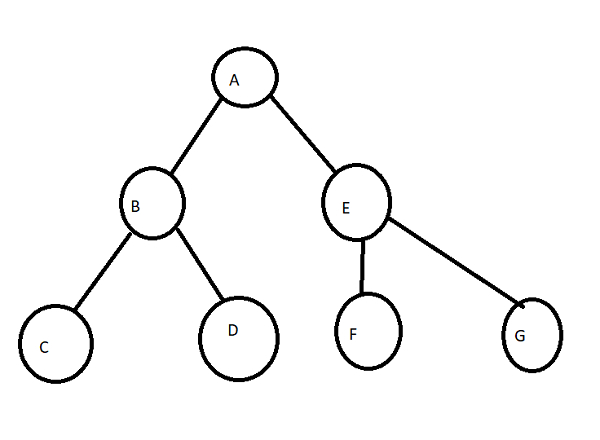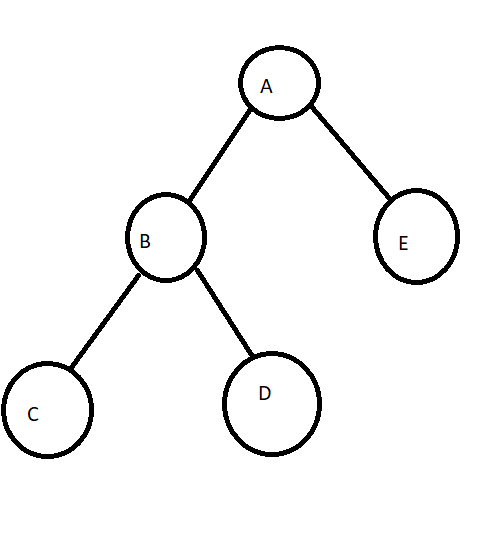# Difference Between B-tree and Binary tree

Computer ProgrammingProgrammingMiscellaneous

In this post, we will understand the difference between B-tree and Binary tree.

## B-tree

• Here, a node can have a maximum of ‘M'(where ‘M’ is the order of the tree) child nodes.

• It is also known as a sorted tree.

• The nodes are present in ‘inorder’ traversal mode.

• It has a height of log M N (where ‘M’ is the order of tree and N is the number of nodes).

• Operations are performed on a B-Tree when data is loaded to the disk.

• It is used in database management system to index code.

• It can also be used to insert the data or key in a B-tree.

• This is a big procedure in comparison to operations on a binary tree.## Binary tree

• Here, a node can have maximum two child nodes (they are also known as sub-trees).

• It is not a sorted tree.

• It can be sorted in inorder, preorder or postorder traversal.

• It has a height of log2 N (base 2, log N, where N is the number of nodes).

• Binary tree operations are used when data is loaded to the RAM (quicker).

• Its applications include usage in Huffman coding.

• It is also used in code optimization methods.

• Data insertion is relatively easier in comparison to B-Tree.